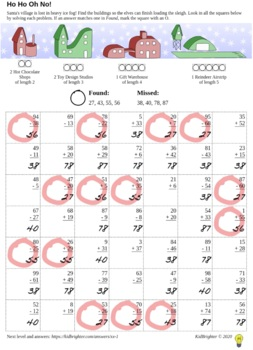# 3rd Grade December Math Worksheets3rd, Homeschool
Subjects
Standards
Resource Type
Formats Included
• PDF
Pages
107 worksheets

### Description

Practice math multiplication, division, addition, subtraction and matching problems while uncovering the north pole village! Over 100 worksheets for all levels of learners in grade 3. Perfect for homework, homeschool, and math center activities.

The worksheets "play" like the classic game of battleships, students solve a problem and then match whether or not the answer indicates a found or empty square.

This package includes many variations including number comparisons, fill in the blanks, and place value practise.

Answers are included to all questions.

Total Pages
107 worksheets
Included
Teaching Duration
N/A
Report this Resource to TpT
Reported resources will be reviewed by our team. Report this resource to let us know if this resource violates TpT’s content guidelines.

### Standards

to see state-specific standards (only available in the US).
Fluently multiply and divide within 100, using strategies such as the relationship between multiplication and division (e.g., knowing that 8 × 5 = 40, one knows 40 ÷ 5 = 8) or properties of operations. By the end of Grade 3, know from memory all products of two one-digit numbers.
Understand division as an unknown-factor problem. For example, find 32 ÷ 8 by finding the number that makes 32 when multiplied by 8.
Determine the unknown whole number in a multiplication or division equation relating three whole numbers. For example, determine the unknown number that makes the equation true in each of the equations 8 × ? = 48, 5 = __ ÷ 3, 6 × 6 = ?.
Interpret whole-number quotients of whole numbers, e.g., interpret 56 ÷ 8 as the number of objects in each share when 56 objects are partitioned equally into 8 shares, or as a number of shares when 56 objects are partitioned into equal shares of 8 objects each. For example, describe a context in which a number of shares or a number of groups can be expressed as 56 ÷ 8.
Multiply one-digit whole numbers by multiples of 10 in the range 10–90 (e.g., 9 × 80, 5 × 60) using strategies based on place value and properties of operations.